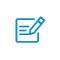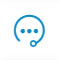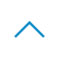# 神经网络详解，正向传播和反向传播• z是x和w、b线性运算的结果，z=wx+b；a是z的激活值；下标的1,2,3,4代表该层的第i个神经元（unit）；上标的,等代表当前是第几层。y^代表模型的输出，y才是真实值，也就是标签

- 上图中的x1，x2，x3，x4不是代表4个样本！而是一个样本的四个特征（4个维度的值）！• 前向传播（Forward Propagation）前向传播就是从input，经过一层层的layer，不断计算每一层的z和a，最后得到输出y^ 的过程，计算出了y^，就可以根据它和真实值y的差别来计算损失（loss）。反向传播（Backward Propagation）反向传播就是根据损失函数L(y^,y)来反方向地计算每一层的z、a、w、b的偏导数（梯度），从而更新参数。
• 前向传播和反向传播：Layer 1: Z = W·X + bA = σ(Z)

Layer 2: Z = W·A + bA = σ(Z)

Layer i: Z[i] = W[i]·A[i-1] + b[i]A[i] = σ(Z[i])

（注：σ是sigmoid函数）

J(W,b) = 1/m·ΣL(y^(i),y(i))• W[l]:(n[l],n[l-1])（该层的单元数，上层的单元数）b[l]:(n[l],1)z[l]:(n[l],1)Z[l]:(n[l],m)a[l]:(n[l],1)A[l]:(n[l],m)X:(n,m)Y:(1,m)扫码入群咨询反馈返回顶部